black nike shoes roshe cheapnicesports.com

# Logic Gates

Logic Gates are building bricks of any digital system. These are the most basic component in digital electronics any one can think of. There are three basic gates, namely AND gate, OR gate and NOT gate. Because of their intuitive name and unique behavior, you will rarely confuse among them. All other gates such as XOR, XNOR, NAND and NOR can be made from the basic ones.

First, lets understand few things common to these gates.

1. These gates can understand only two voltage levels, 0 volt and 5 volts. In general discussion, 0V is called logic '0', and 5V is called logic '1'. The output generated by these gates are also in terms of logic '0' or logic '1'. i.e the output generated by any gate can be either 0V or 5V.
2. At a time only logic '0' or logic '1' can be fed to a input pin. Similarly any output pin can have only 0V or 5V at a time (logic 0 or logic 1).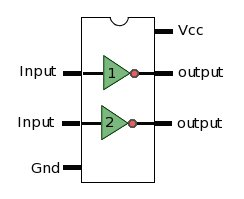3. Generally multiple such gates are fabricated in on IC (e.g 4). Power and ground pins for these ICs are separate, don't get confused that how a NOT gate is generating 5V at the output when 0V is supplied at input ?
4. All GATEs can have multiple inputs, but will have only one output. For example 4 input AND gate, has 4 input pins and one output.
5. A NOT gate has only one input and one output. NOT gates cannot have more than one input.
6. GATE's behavior for different inputs are represented in a table, this table is called TRUTH TABLE . These TRUTH TABLE represents output behavior for all combination of input.
7. These GATES can also be represented in terms of boolean expression. where AND GATE is represented by . (period), OR GATE by + (plus) and NOT GATE by ¯ (a bar above expression). For example a two input AND GATE can be represented as (A AND B) where A,B are inputs of a two input AND GATE.
8. This boolean expressions are very convenient since they help in representing any GATE circuit in simple expression.

Below showing few gates, their symbol, boolean expression and truth Table...

AND GATE

AND Gate Boolean Expression TRUTH Table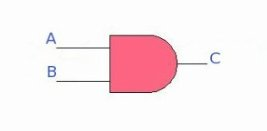C = A.B
 A B C (Out) 0 0 0 0 1 0 1 0 0 1 1 1

OR GATE

OR Gate Boolean Expression TRUTH Table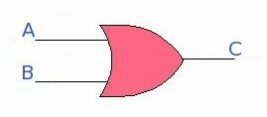C = A + B
 A B C (Out) 0 0 0 0 1 1 1 0 1 1 1 1

XOR GATE

XOR Gate Boolean Expression TRUTH Table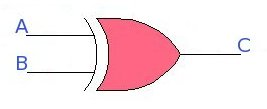C = A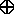B
```       Or
_   _
C = A.B + A.B
```
 A B C (Out) 0 0 0 0 1 1 1 0 1 1 1 0

NOTE : From the boolean expression it is clear that XOR gate is can be made by using NOT, AND, and OR basic gates.

NAND GATE

NAND Gate Boolean Expression TRUTH Table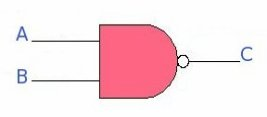```    ___
C = A.B
```
 A B C (Out) 0 0 1 0 1 1 1 0 1 1 1 0

NOTE : A NAND gate is just a NOT gate in front of AND gate. Hence the bubble in front of NAND is a NOT gate itself.

NOR GATE

NOR Gate Boolean Expression TRUTH Table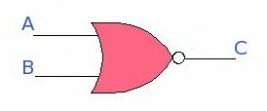```    _____
C = A + B
```
 A B C (Out) 0 0 1 0 1 0 1 0 0 1 1 0

NOTE : A NOR gate is just a NOT gate in front of OR gate. Hence the bubble in front of OR is a NOT gate itself.

XNOR GATE

XNOR Gate Boolean Expression TRUTH TableC = A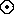B
```       Or
_________
-   -
C = A.B + A.B
```
 A B C (Out) 0 0 1 0 1 0 1 0 0 1 1 1

NOTE : A XNOR gate is just a NOT gate in front of XOR gate. Hence the bubble in front of OR is a NOT gate itself.

NOT GATE

NOT Gate Boolean Expression TRUTH Table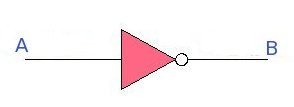```     _
B = A
```
 A B (Out) 0 1 1 0